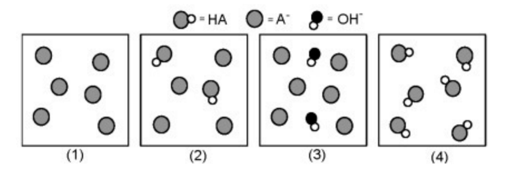# Problem: The following pictures represent solutions at various points in the titration of a weak acid HA with aqueous KOH. Unshaded spheres represent H atoms, black spheres represent oxygen atoms, and shaded spheres represent A- ions. (K+, H3O+, initially present, OH - initially present, and solvent H2O molecules have been omitted for clarity). Which picture below represents the solution at the equivalence point? (a) 1 (b) 2 (c) 3 (d) 4 (e) None of the above.

###### FREE Expert Solution
95% (270 ratings)
###### Problem Details

The following pictures represent solutions at various points in the titration of a weak acid HA with aqueous KOH. Unshaded spheres represent H atoms, black spheres represent oxygen atoms, and shaded spheres represent A- ions. (K+, H3O+, initially present, OH - initially present, and solvent H2O molecules have been omitted for clarity).

Which picture below represents the solution at the equivalence point?

(a) 1

(b) 2

(c) 3

(d) 4

(e) None of the above.What scientific concept do you need to know in order to solve this problem?

Our tutors have indicated that to solve this problem you will need to apply the Equivalence Point concept. If you need more Equivalence Point practice, you can also practice Equivalence Point practice problems.

What is the difficulty of this problem?

Our tutors rated the difficulty ofThe following pictures represent solutions at various points...as medium difficulty.

How long does this problem take to solve?

Our expert Chemistry tutor, Jules took 2 minutes and 51 seconds to solve this problem. You can follow their steps in the video explanation above.

What professor is this problem relevant for?

Based on our data, we think this problem is relevant for Professor Schreiber's class at WMU.